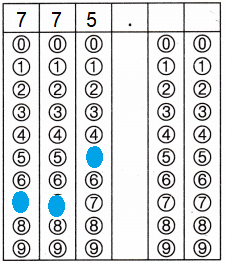Refer to our Texas Go Math Grade 4 Answer Key Pdf to score good marks in the exams. Test yourself by practicing the problems from Texas Go Math Grade 4 Module 11 Assessment Answer Key.

Concepts and Skills

Find the sum.

Question 1.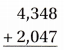Explanation:
An addition sentence is a mathematical expression that shows two or more values added together to find their sum.

Question 2.Explanation:
An addition sentence is a mathematical expression that shows two or more values added together to find their sum.

Question 3.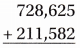Explanation:
An addition sentence is a mathematical expression that shows two or more values added together to find their sum.

Find the value of n.

Question 4.
264,185 + 38,642 = n
____________ = n
Explanation:
An addition sentence is a mathematical expression that shows two or more values added together to find their sum.
264,185 + 38,642 = n
n = 302,642

Question 5.
238,375 + 29,558 = n
____________ = n
Explanation:
An addition sentence is a mathematical expression that shows two or more values added together to find their sum.
238,375 + 29,558 = n
n = 267,933

Question 6.
342,205 + 127,539 = n
____________ = n
Explanation:
An addition sentence is a mathematical expression that shows two or more values added together to find their sum.
342,205 + 127,539 = n
n = 469,744

Find the difference.

Question 7.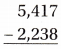Explanation:
Subtraction is an arithmetic operation that represents the operation of removing objects from a collection.
Subtraction is signified by the minus sign(−)

Question 8.Explanation:
Subtraction is an arithmetic operation that represents the operation of removing objects from a collection.
Subtraction is signified by the minus sign(−)

Question 9.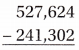Explanation:
Subtraction is an arithmetic operation that represents the operation of removing objects from a collection.
Subtraction is signified by the minus sign(−)

Find the value of n.

Question 10.
162,712 – 24,729 = n
____________ = n
Explanation:
Subtraction is an arithmetic operation that represents the operation of removing smaller number from bigger number.
Subtraction is signified by the minus sign(−)
162,712 – 24,729 = n
n = 137,983

Question 11.
483,700 – 26,308 = n
____________ = n
Explanation:
Subtraction is an arithmetic operation that represents the operation of removing smaller number from bigger number.
Subtraction is signified by the minus sign(−)
483,700 – 26,308 = n
n = 457,392

Question 12.
400,000 – 128,803 = n
____________ = n
Explanation:
Subtraction is an arithmetic operation that represents the operation of removing smaller number from bigger number.
Subtraction is signified by the minus sign(−)
400,000 – 128,803 = n
n = 271,197

Find the Product.

Question 13.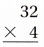Explanation:
When two or more values multiplied together to get answer is called product.

Question 14.Explanation:
When two or more values multiplied together to get answer is called product.

Question 15.Explanation:
When two or more values multiplied together to get answer is called product.

Question 16.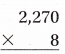Explanation:
When two or more values multiplied together to get answer is called product.

Find the Value of n.

Question 17.
24 ×10 = n
____________ = n
Explanation:
To find the n value, add a number together as many times as the number is multiplied by.
24 ×10 = n
24 is added for ten times to get the product.
So, n = 240

Question 18.
100 × 36 = n
____________ = n
Explanation:
To find the n value, add a number together as many times as the number is multiplied by.
100 ×36 = n
100 is added for 36 times to get the product.
So, n = 3600

Question 19.
45 × 23 = n
____________ = n
Explanation:
To find the n value, add a number together as many times as the number is multiplied by.
45 ×23 = n
45 is added for 23 times to get the product.
So, n = 1,035

Find the value of n.

Question 20.
65 ÷ 5 = n
____________ = n
Explanation:
The division is a method of distributing a group of things into equal parts.
It is one of the four basic operations of arithmetic, which gives a fair result of sharing.
65 ÷ 5 = n
n = 13

Question 21.
516 ÷ 3 = n
____________ = n
Explanation:
The division is a method of distributing a group of things into equal parts.
It is one of the four basic operations of arithmetic, which gives a fair result of sharing.
516 ÷ 3 = n
n = 172

Question 22.
620 ÷ 4 = n
____________ = n
Explanation:
The division is a method of distributing a group of things into equal parts.
It is one of the four basic operations of arithmetic, which gives a fair result of sharing.
620 ÷ 4 = n
n = 155

Question 23.
1,026 ÷ 9 = n
____________ = n
Explanation:
The division is a method of distributing a group of things into equal parts.
It is one of the four basic operations of arithmetic, which gives a fair result of sharing.
1,026 ÷ 9 = n
n = 114

Question 24.
During October, Joe’s ice cream shop had 11,094 customers and Tatum’s yogurt shop had 10,237 customers. Matt’s yogurt shop had 3,810 more customers than Tatum’s shop. How many customers did all three shops have during October?
(A) 14,047
(B) 5,640
(C) 35,378
(D) 22,331
Option(C)
Explanation:
During October, Joe’s ice cream shop had 11,094 customers and
Tatum’s yogurt shop had 10,237 customers.
Matt’s yogurt shop had 3,810 more customers than Tatum’s shop.
Total customers of Matt’s yogurt shop 3810 + 10237 = 14,047
Total customers in all three shops have during October
11,094 + 10,237 + 14,047 = n
n = 35,378

Question 25.
The town of Summerville has a population of 40,285 during the summer, a population of 18,463 during the winter, and a population of 38,709 during the fall. How many more people spend time in Sum merville during the winter and fall combined than during the summer?
(A) 20,246
(B) 57,172
(C) 7,501
(D) 16,887
Option(D)
Explanation:
The town of Summerville has a population of 40,285 during the summer,
A population of 18,463 during the winter, and
A population of 38,709 during the fall.
Number of more people spend time in Summerville during the winter and fall combined than during the summer
(18,463 + 38,709) – 40,285  = n
57,172 – 40,285 = n
16,887 = n

Question 26.
The Davis family are on a ten day road trip. They travel 10 hours each day for 3 days. They travel 8 hours each day for 7 days. How many hours does the Davis family travel during their road trip?
(A) 86
(B) 56
(C) 26
(D) 30
Option(A)
Explanation:
The Davis family travel 10 hours each day for 3 days.
10 x 3 = 30 hours.
They travel 8 hours each day for 7 days.
8 x 7 = 56 hours.
Total hours does the Davis family travel during their road trip
(10 x 3) + (8 x 7) = n
30 + 56 = n
86 = n

Question 27.
The garden warehouse delivered 1,550 pounds of topsoil in 5-pound bags to the garden shop. The garden shop sold half of the bags the same day they were delivered. How many bags does the garden shop have left to sell?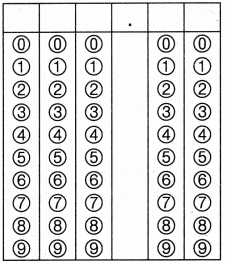Record your answer and fill in the bubbles on the grid. Be sure to use the correct place value.